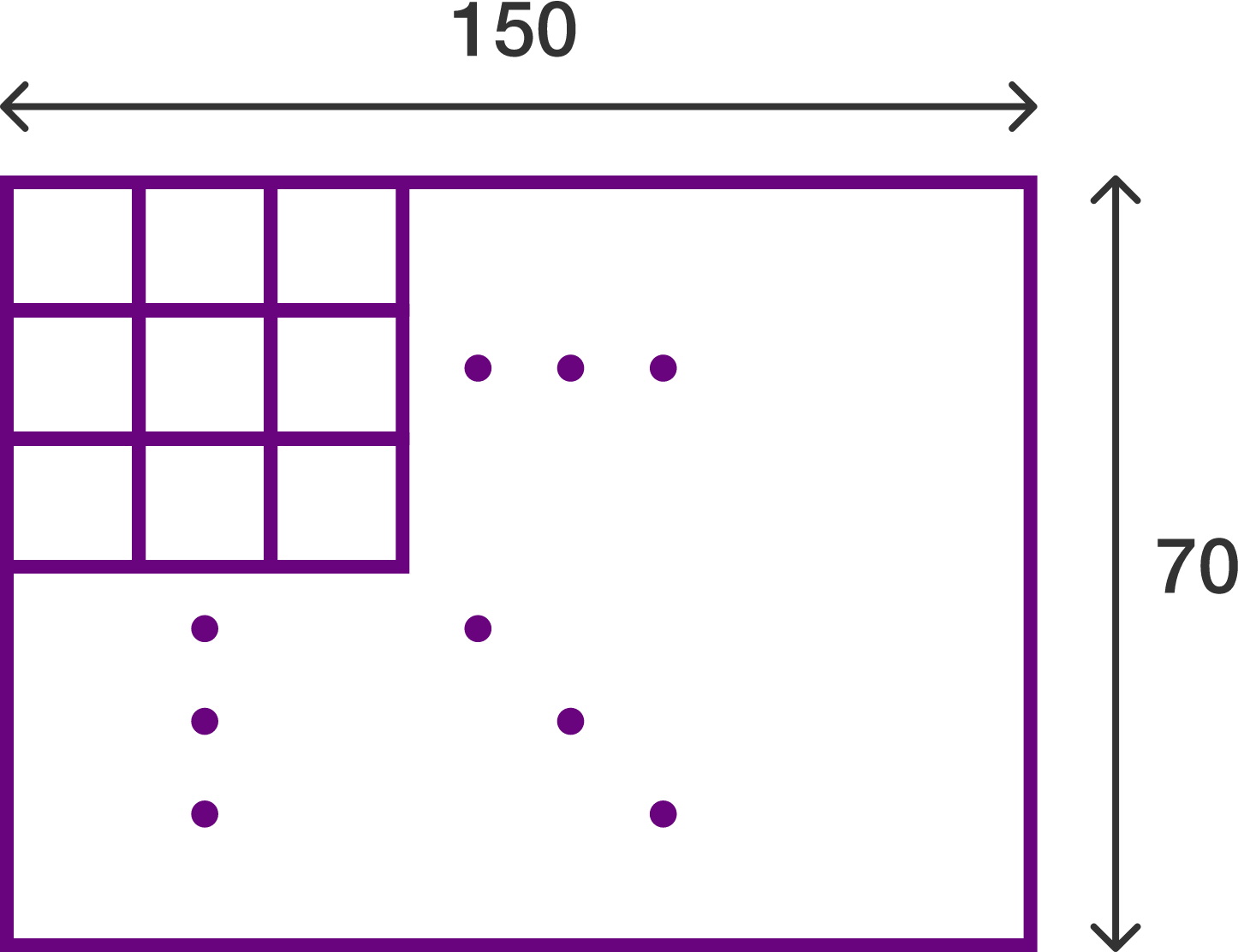# Picky Rectangles

Probability Level 5A $150\times 70$ grid has $178\ x\times y$ rectangles. Find the value of $x+y$.

Details and Assumptions:

• Dimensions are expressed as width$\times$height. Thus an $x\times y$ rectangle (that is, a rectangle of width $x$ and height $y$) is considered to be different from a $y\times x$ (width $y,$ height $x$) rectangle. In this problem, you only consider the $x\times y$ rectangles, not the $y\times x$ ones.

×